# Lecture 2

## Welcome!

• In our previous session, we learned about C, a text-based programming language.
• This week, we are going to take a deeper look at additional building-blocks that will support our goals of learning more about programming from the bottom up.
• Fundamentally, in addition to the essentials of programming, this course is about problem-solving. Accordingly, we will also focus further on how to approach computer science problems.

## Compiling

• Encryption is the act of hiding plain text from prying eyes. decrypting, then, is the act of taking an encrypted piece of text and returning it to a human-readable form.
• An encrypted piece of text may look like the following: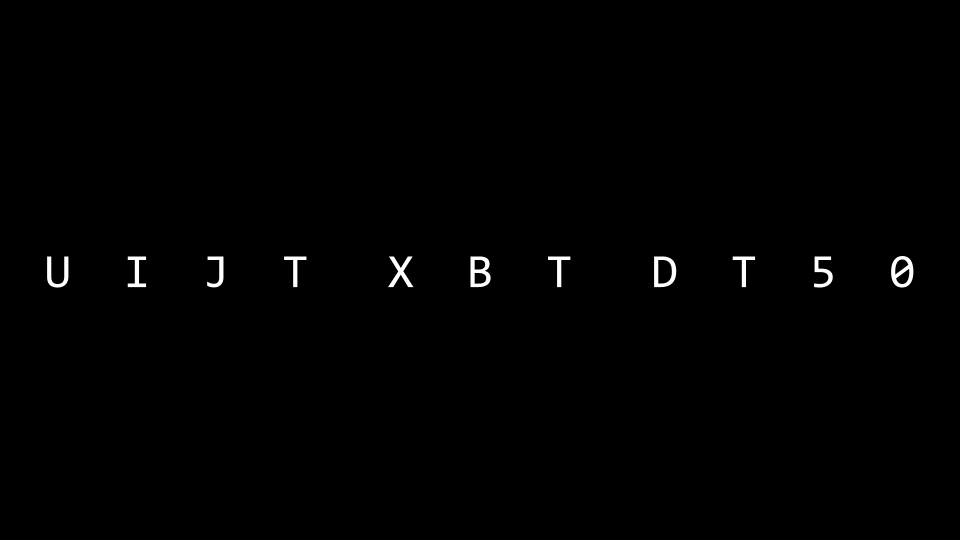• Recall that last week you learned about a compiler, a specialized computer program that converts source code into machine code that can be understood by a computer.
• For example, you might have a computer program that looks like this:

#include <stdio.h>

int main(void)
{
printf("hello, world\n");
}

• A compiler will take the above code and turn it into the following machine code: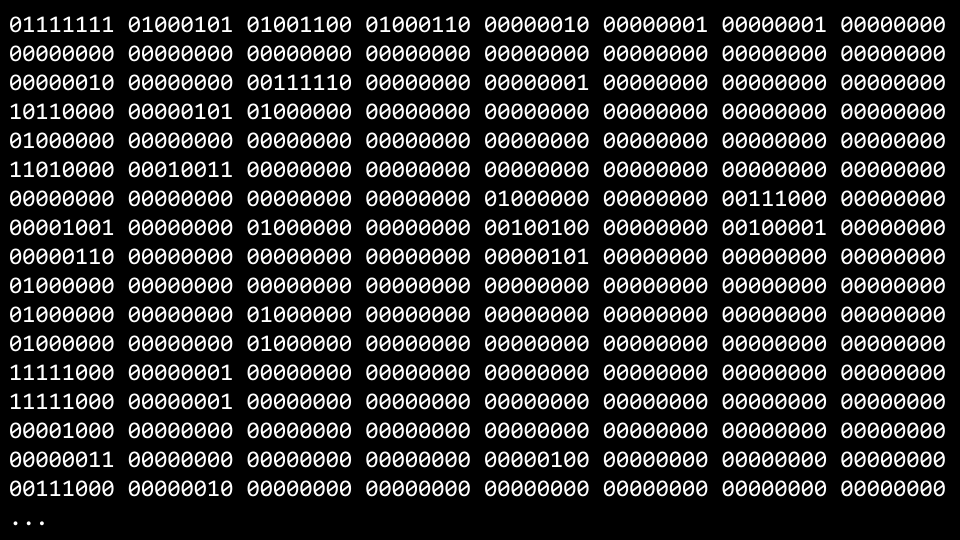• VS Code, the programming environment provided to you as a CS50 student, utilizes a compiler called clang or c language.
• If you were to type make hello, it runs a command that executes clang to create an output file that you can run as a user.
• VS Code has been pre-programmed such that make will run numerous command line arguments along with clang for your convenience as a user.
• Consider the following code:

#include <cs50.h>
#include <stdio.h>

int main(void)
{
string name = get_string("What's your name? ");
printf("hello, %s\n", name);
}

• You can attempt to enter into the terminal window: clang -o hello hello.c. You will be met by an error that indicates that clang does not know where to find the cs50.h library.
• Attempting again to compile this code, run the following command in the terminal window: clang -o hello hello.c -lcs50. This will enable the compiler to access the cs50.h library.
• Running in the terminal window ./hello, your program will run as intended.
• While the above is offered as an illustration, such that you can understand more deeply the process and concept of compiling code, using make in CS50 is perfectly fine and the expectation!
• Compiling involves major steps, including the following:
• First, preprocessing is where the header files in your code, designated by a # (such as #include \<cs50.h\>) are effectively copied and pasted into your file. During this step, the code from cs50.h is copied into your program. Similarly, just as your code contains #include \<stdio.h\>, code contained within stdio.h somewhere on your computer is copied to your program. This step can be visualized as follows:
...
string get_string(string prompt);
int printf(string format, ...);
...

int main(void)
{
string name = get_string("What's your name? ");
printf("hello, %s\n", name);
}

• Second, compiling is where your program is converted into assembly code. This step can be visualized as follows: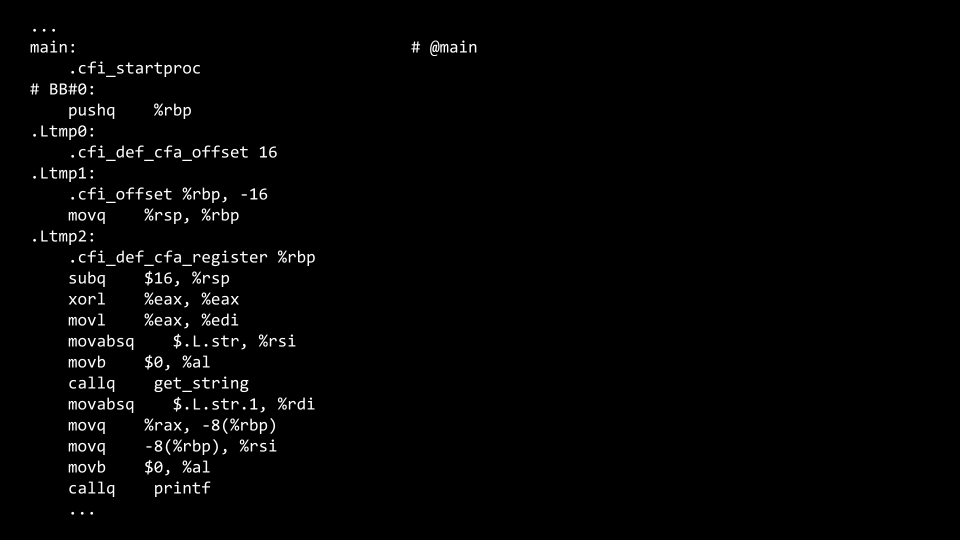• Third, assembling involves the compiler converting your assembly code into machine code. This step can be visualized as follows: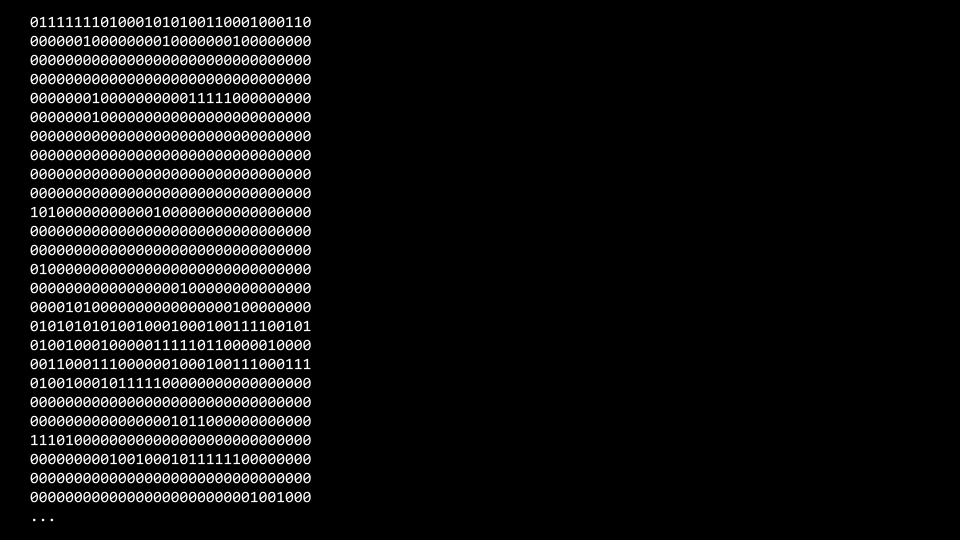• Finally, during the linking step, code from your included libraries are converted also into machine code and combined with your code. The final executable file is then outputted.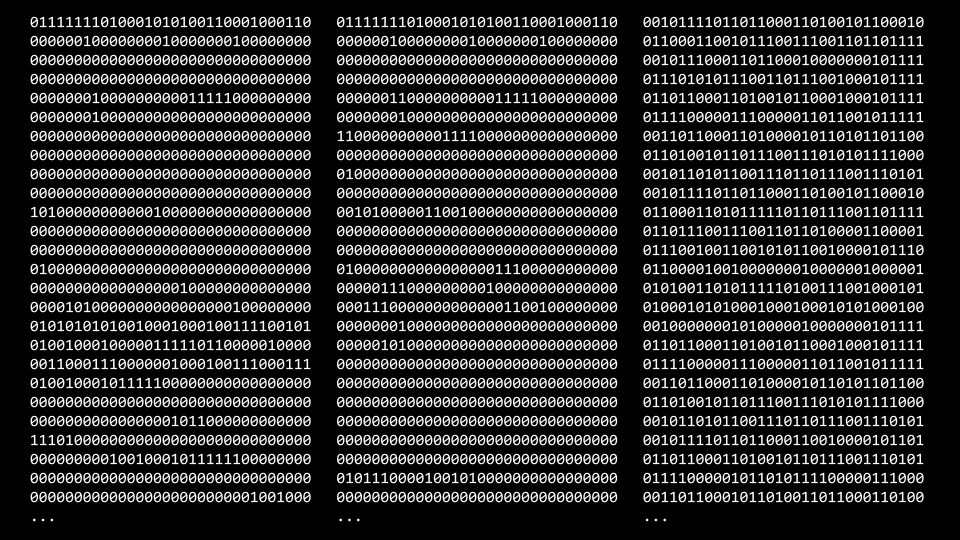## Debugging

• Everyone will make mistakes while coding.
• Consider the following image from last week:• Further, consider the following code that has a bug purposely inserted within it:

#include <stdio.h>

int main(void)
{
for (int i = 0; i <= 3; i++)
{
printf("#\n");
}
}

• Type code buggy0.c into the terminal window and write the above code.
• Running this code, four bricks appear instead of the intended three.
• printf is a very useful way of debugging your code. You could modify your code as follows:

#include <stdio.h>

int main(void)
{
for (int i = 0; i <= 3; i++)
{
printf("i is %i\n", i);
printf("#\n");
}
}

• Running this code, you will see numerous statements, including i is 0, i is 1, i is 2, and i is 3. Seeing this, you might realize that Further code needs to be corrected as follows:

#include <stdio.h>

int main(void)
{
for (int i = 0; i < 3; i++)
{
printf("#\n");
}
}


Notice the <= has been replaced with <.

• A second tool in debugging is called a debugger, a software tool created by programmers to help track down bugs in code.
• In VS Code, a preconfigured debugger has been provided to you.
• To utilize this debugger, first set a breakpoint by clicking to the left of a line of your code, just to the left of the line number. When you click there, you will see a red dot appearing. Imagine this as a stop sign, asking the compiler to pause such that you can consider what’s happening in this part of your code.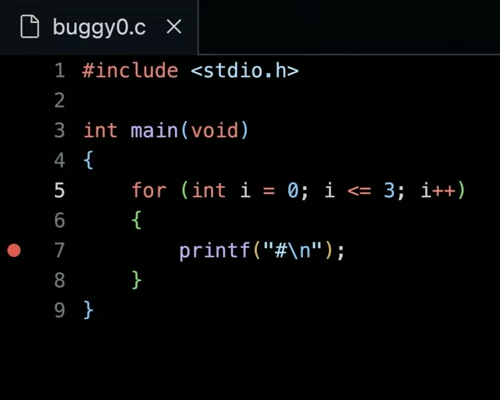• Second, run debug50 ./buggy0. You will notice that after the debugger comes to life that a line of your code will illuminate in a gold-like color. Quite literally, the code has paused at this line of code. Notice in the top left corner how all local variables are being displayed, including i, which has a current value of 0. At the top of your window, you can click the step over button and it will keep moving through your code. Notice how the value of i increases.
• While this tool will not show you where your bug is, it will help you slow down and see how your code is running step by step.

• To illustrate a third means of debugging, please start a new file by running code buggy1.c in your terminal window. Write your code as follows:

#include <cs50.h>
#include <stdio.h>

int get_negative_int(void);

int main(void)
{
int i = get_negative_int();
printf("%i\n", i);
}

// Prompt user for positive integer
int get_negative_int(void)
{
int n;
do
{
n = get_int("Negative Integer: ");
}
while (n < 0);
return n;
}


Notice get_negative_int is designed to get a negative integer from the user.

• Running make buggy1, you’ll notice that it does not do as intended. It accepts positive integers and seems to ignore negative ones.
• As before, set a breakpoint at a line of your code. Best to set a breakpoint at int i = get_negative_int. Now, run debug50 buggy1.
• Unlike before, where you utilized the step over button at the top of the window, use the step into button. This will take the debugger into your get_negative_int function. Notice how doing this will show you that you are, indeed, stuck in the do while loop.
• With this knowledge, you might consider why you are stuck in this loop, leading you to edit your code as follows:

#include <cs50.h>
#include <stdio.h>

int get_negative_int(void);

int main(void)
{
int i = get_negative_int();
printf("%i\n", i);
}

// Prompt user for positive integer
int get_negative_int(void)
{
int n;
do
{
n = get_int("Negative Integer: ");
}
while (n >= 0);
return n;
}


Notice n < 0 has been changed.

• A final form of debugging is called rubber duck debugging. When you are having challenges with your code, consider how speaking out loud to, quite literally, a rubber duck about the code problem. If you’d rather not talk to a small plastic duck, you are welcome to speak to a human near you! They need not understand how to program: Speaking with them is an opportunity for you to speak about your code.## Arrays

• In Week 0, we talked about data types such as bool, int, char, string, etc.
• Each data type requires a certain amount of system resources:
• bool 1 byte
• int 4 bytes
• long 8 bytes
• float 4 bytes
• double 8 bytes
• char 1 byte
• string ? bytes
• Inside of your computer, you have a finite amount of memory available.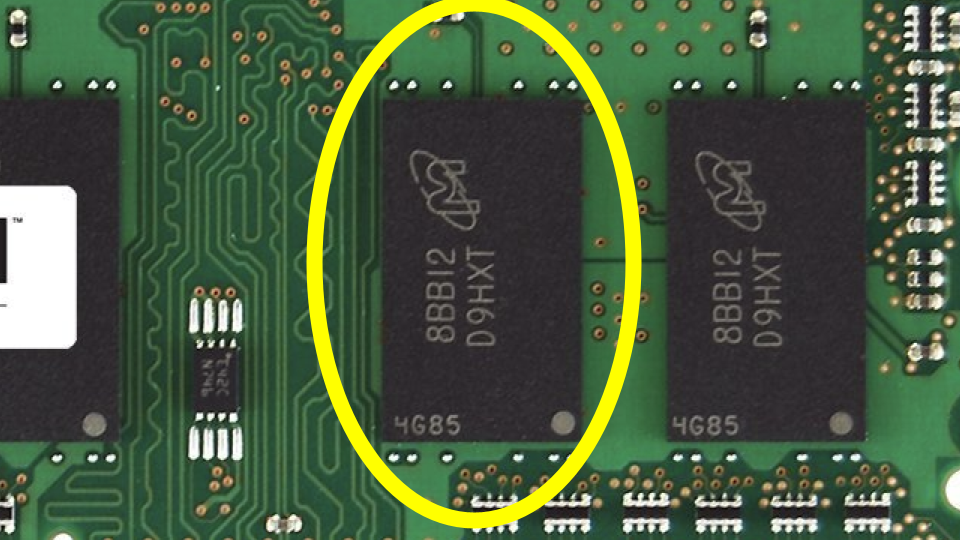• Physically, on the memory of your computer, you can imagine how specific types of data are stored on your computer. You might imagine that a char, which only requires 1 byte of memory, may look as follows: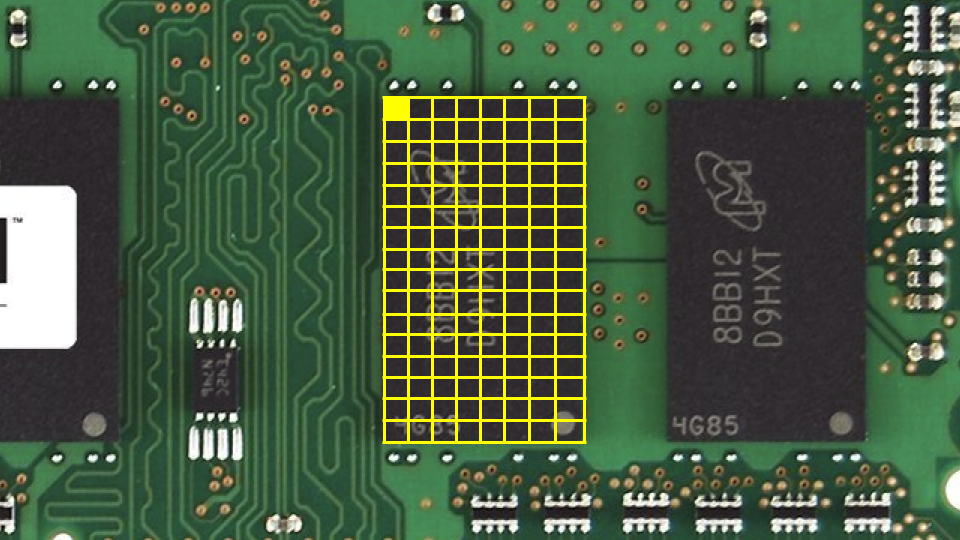• Similarly, an int, which requires 4 bytes might look as follows: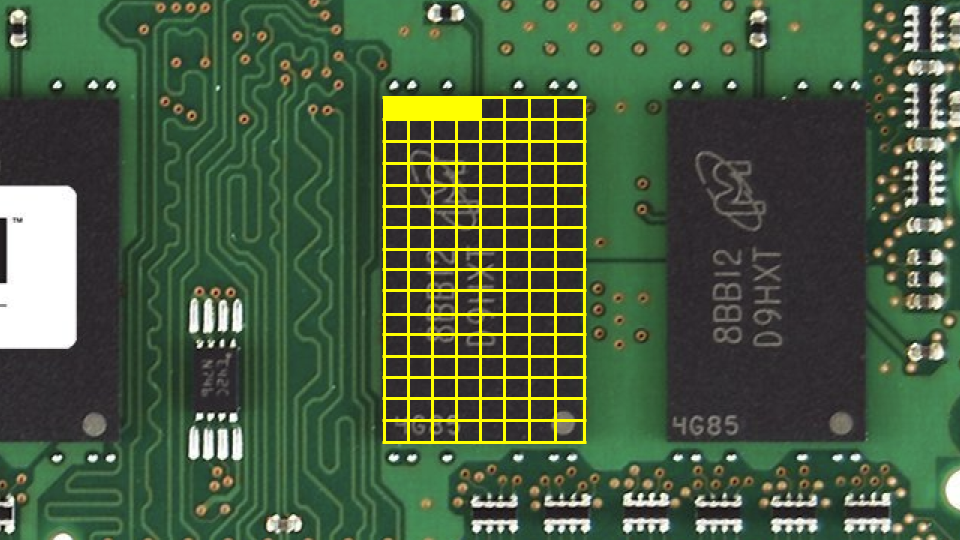• We can create a program that explores these concepts. Inside your terminal, type code scores.c and write code as follows:

#include <stdio.h>

int main(void)
{
// Scores
int score1 = 72;
int score2 = 73;
int score3 = 33;

// Print average
printf("Average: %f\n", (score1 + score2 + score3) / 3.0);
}


Notice that the number on the right is a floating point value of 3.0, such that the calculation is rendered as a floating point value in the end.

• Running make scores, the program runs.
• You can imagine how these variables are stored in memory: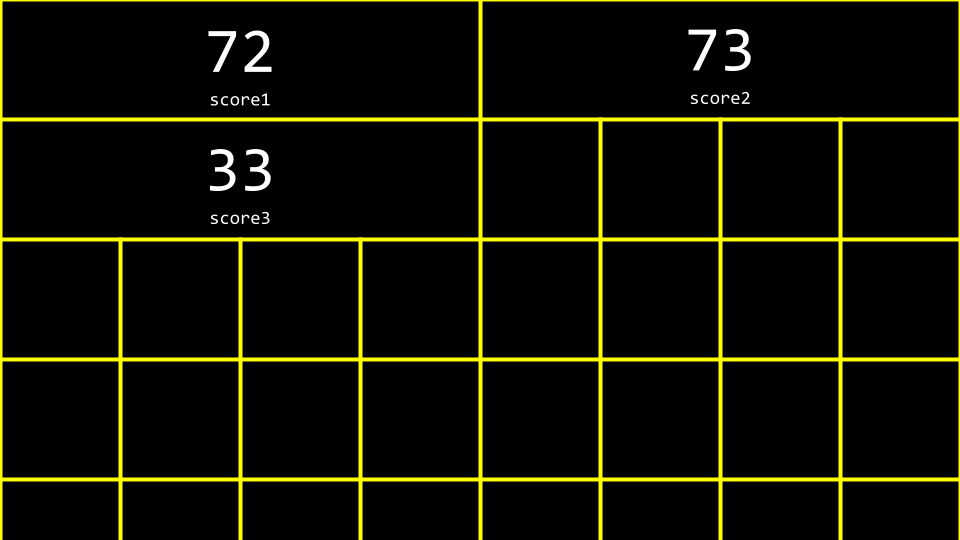• Arrays are a way of storing data back-to-back in memory such that this data is easily accessible.
• int scores is a way of telling the compiler to provide you three back-to-back places in memory of size int to store three scores. Considering our program, you can revise your code as follows:

#include <cs50.h>
#include <stdio.h>

int main(void)
{
// Scores
int scores;
scores = 72;
scores = 73;
scores = 33;

// Print average
printf("Average: %f\n", (scores + scores + scores) / 3.0);
}


Notice that score examines the value at this location of memory by indexing into the array called scores at location 0 to see what value is stored there.

• You can see how while the above code works, there is still an opportunity for improving our code. Revise your code as follows:

#include <cs50.h>
#include <stdio.h>

int main(void)
{
// Get scores
int scores;
for (int i = 0; i < 3; i++)
{
scores[i] = get_int("Score: ");
}

// Print average
printf("Average: %f\n", (scores + scores + scores) / 3.0);
}


Notice how we index into scores by using scores[i] where i is supplied by the for loop.

• We can simplify or abstract away the calculation of the average. Modify your code as follows:

#include <cs50.h>
#include <stdio.h>

// Constant
const int N = 3;

// Prototype
float average(int length, int array[]);

int main(void)
{
// Get scores
int scores[N];
for (int i = 0; i < N; i++)
{
scores[i] = get_int("Score: ");
}

// Print average
printf("Average: %f\n", average(N, scores));
}

float average(int length, int array[])
{
// Calculate average
int sum = 0;
for (int i = 0; i < length; i++)
{
sum += array[i];
}
return sum / (float) length;
}


Notice that a new function called average is declared. Further, notice that a const or constant value of N is declared. Most importantly, notice how the average function takes int array[], which means that the compiler passes an array to this function.

• Not only can arrays be containers: They can be passed between functions.

## Strings

• A string is simply an array of variables of type char: an array of characters.
• Considering the following image, you can see how a string is an array of characters that begins with the first character and ends with a special character called a NUL character: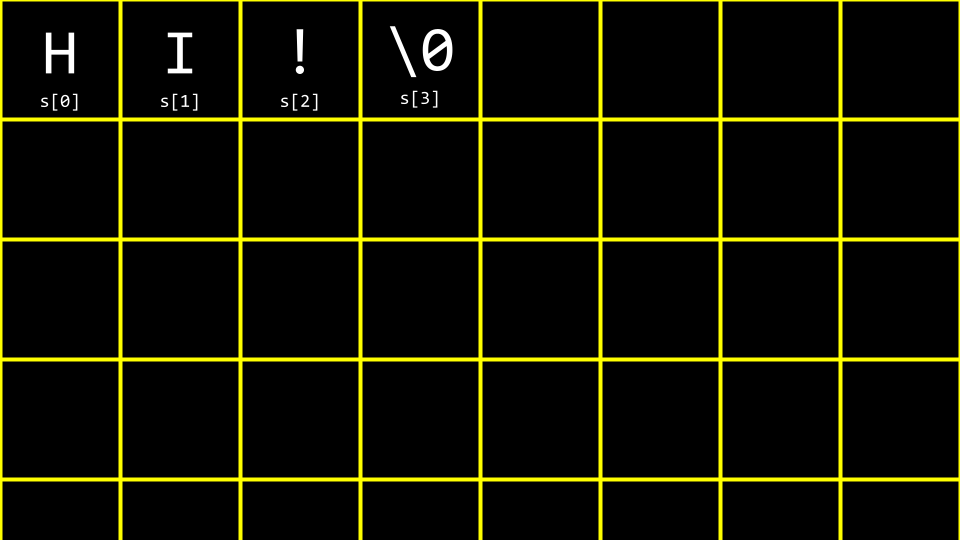• Imagining this in decimal, your array would look like the following: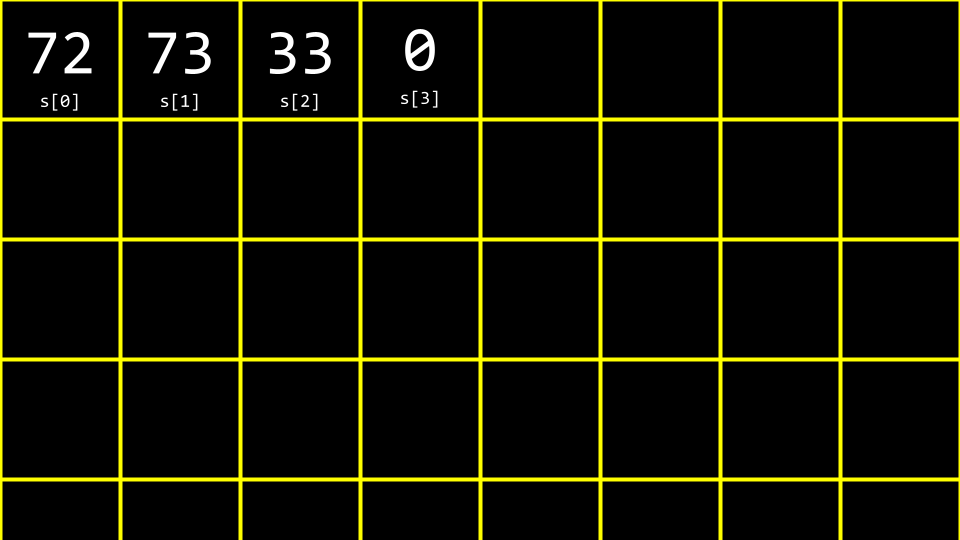• Implementing this in your own code, type code hi.c in the terminal window and write code as follows:

#include <stdio.h>

int main(void)
{
char c1 = 'H';
char c2 = 'I';
char c3 = '!';

printf("%i %i %i\n", c1, c2, c3);
}


Notice that this will output the decimal values of each character.

• To further understand how a string works, revise your code as follows:

#include <cs50.h>
#include <stdio.h>

int main(void)
{
string s = "HI!";
printf("%i %i %i\n", s, s, s);
}


Notice how the printf statement presents three values from our array called s.

• Let’s imagine we want to say both HI! and BYE!. Modify your code as follows:

#include <cs50.h>
#include <stdio.h>

int main(void)
{
string s = "HI!";
string t = "BYE!";

printf("%s\n", s);
printf("%s\n", t);
}


Notice that two strings are declared and used in this example.

• You can visualize this as follow: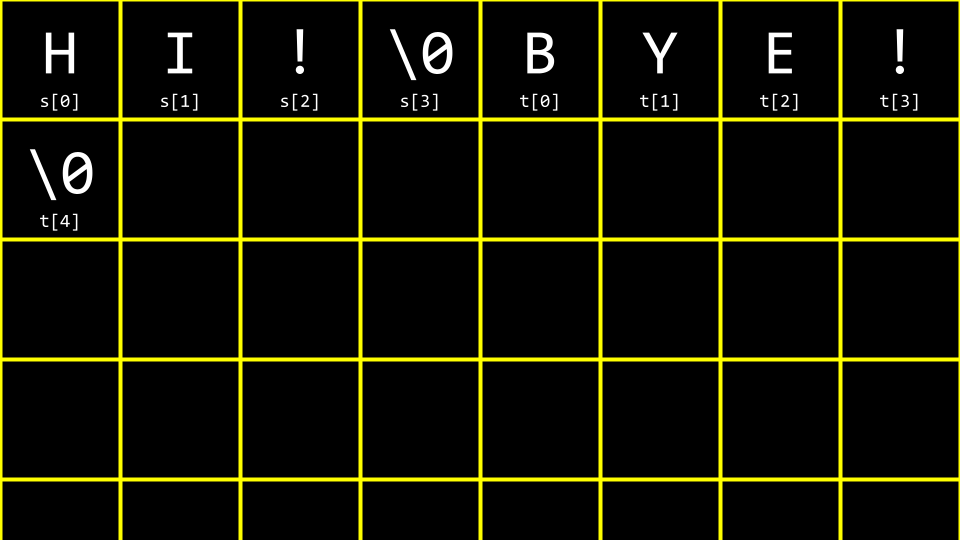• We can further improve this code. Modify your code as follows:

#include <cs50.h>
#include <stdio.h>

int main(void)
{
string words;

words = "HI!";
words = "BYE!";

printf("%s\n", words);
printf("%s\n", words);
}


Notice that both strings are stored within a single array of type string.

• A common problem within programming, and perhaps C more specifically, is to discover the length of an array. How could we implement this in code? Type code length.c in the terminal window and code as follows:

#include <cs50.h>
#include <stdio.h>

int main(void)
{
// Prompt for user's name
string name = get_string("Name: ");

// Count number of characters up until '\0' (aka NUL)
int n = 0;
while (name[n] != '\0')
{
n++;
}
printf("%i\n", n);
}


Notice that this code loops until the NUL character is found.

• Since this is such a common problem within programming, other programmers have created code in the string.h library to find the length of a string. You can find the length of a string by modifying your code as follows:

#include <cs50.h>
#include <stdio.h>
#include <string.h>

int main(void)
{
// Prompt for user's name
string name = get_string("Name: ");
int length = strlen(name);
printf("%i\n", length);
}


Notice that this code uses the string.h library, declared at the top of the file. Further, it uses a function from that library called strlen, which calculates the length of the string passed to it.

• ctype.h is another library that is quite useful. Imagine we wanted to create a program that converted all lowercase characters to uppercase ones. In the terminal window type code uppercase.c and write code as follows:

#include <cs50.h>
#include <stdio.h>
#include <string.h>

int main(void)
{
string s = get_string("Before: ");
printf("After:  ");
for (int i = 0, n = strlen(s); i < n; i++)
{
if (s[i] >= 'a' && s[i] <= 'z')
{
printf("%c", s[i] - 32);
}
else
{
printf("%c", s[i]);
}
}
printf("\n");
}


Notice that this code iterates through each value in the string. The program looks at each character. If the character is lowercase, it subtracts the value 32 from it to convert it to lowercase.

• Recalling our previous work from last week, you might remember this ASCII values chart: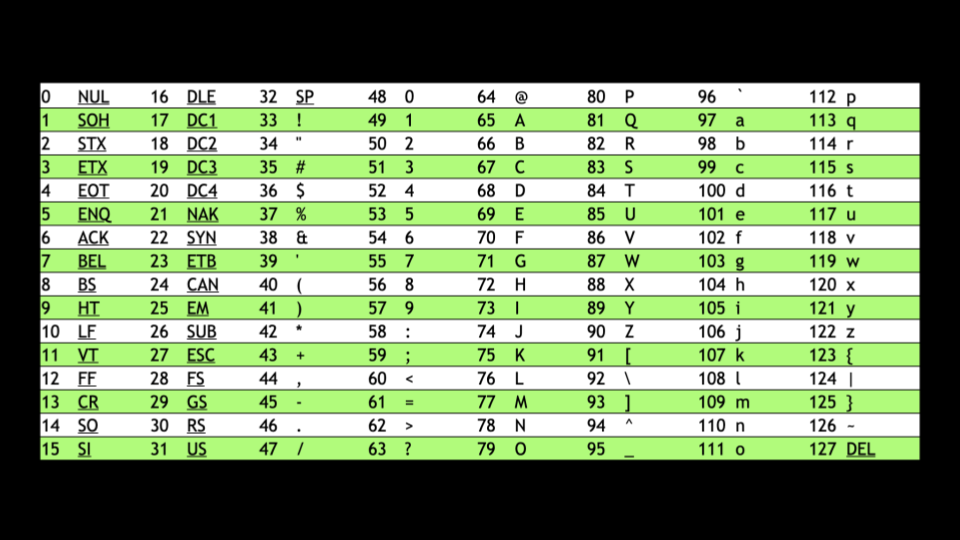• When a lowercase character has 32 subtracted from it, it results in an uppercase version of that same character.
• While the program does what we want, there is an easier way using the ctype.h library. Modify your program as follows:

#include <cs50.h>
#include <ctype.h>
#include <stdio.h>
#include <string.h>

int main(void)
{
string s = get_string("Before: ");
printf("After:  ");
for (int i = 0, n = strlen(s); i < n; i++)
{
if (islower(s[i]))
{
printf("%c", toupper(s[i]));
}
else
{
printf("%c", s[i]);
}
}
printf("\n");
}


Notice that the program uses islower to detect if each character is uppercase or lowercase. Then, the toupper function is passed s[i]. Each character (if lowercase) is converted to uppercase.

• Again, while this program does what is desired, there is an opportunity for improvement. As the documentation for ctype.h states, toupper is smart enough to know that if it is passed what is already an uppercase letter, it will simply ignore it. Therefore, we no longer need our if statement. You can simplify this code as follows:

#include <cs50.h>
#include <ctype.h>
#include <stdio.h>
#include <string.h>

int main(void)
{
string s = get_string("Before: ");
printf("After:  ");
for (int i = 0, n = strlen(s); i < n; i++)
{
printf("%c", toupper(s[i]));
}
printf("\n");
}


Notice that this code is quite simplified, removing the unnecessary if statement.

• You can read about all the capabilities of the ctype library on the Manual Pages.

## Command-Line Arguments

• Command-line arguments are those arguments that are passed to your program at the command line. For example, all those statements you typed after clang are considered command line arguments. You can use these arguments in your own programs!
• In your terminal window, type code greet.c and write code as follows:

#include <cs50.h>
#include <stdio.h>

int main(void)
{
string name = get_string("What's your name? ");
printf("hello, %s\n", name);
}


Notice that this says hello to the user.

• Still, would it not be nice to be able to take arguments before the program even runs? Modify your code as follows:

#include <cs50.h>
#include <stdio.h>

int main(int argc, string argv[])
{
if (argc == 2)
{
printf("hello, %s\n", argv);
}
else
{
printf("hello, world\n");
}
}


Notice that this program knows both argc, the number of command line arguments, and argv which is an array of the characters passed as arguments at the command line.

• Therefore, using the syntax of this program, executing ./greet David would result in the program saying hello, David.

## Exit Status

• When a program ends, a special exit code is provided to the computer.
• When a program exits without error, a status code of 0 is provided the computer. Often, when an error occurs that results in the program ending, a status of 1 is provided by the computer.
• You could write a program as follows that illustrates this by typing code status.c and writing code as follows:

#include <cs50.h>
#include <stdio.h>

int main(int argc, string argv[])
{
if (argc != 2)
{
printf("Missing command-line argument\n");
return 1;
}
printf("hello, %s\n", argv);
return 0;
}


Notice that if you fail to provide ./status David, you will get an exit status of 1. However, if you do provide ./status David, you will get an exit status of 0.

• You can imagine how you might use portions of the above program to check if a user provided the correct number of command-line arguments.

## Cryptography

• Cryptography is the art of ciphering and deciphering a message.
• plaintext and a key are provided to a cipher, resulting in ciphered text.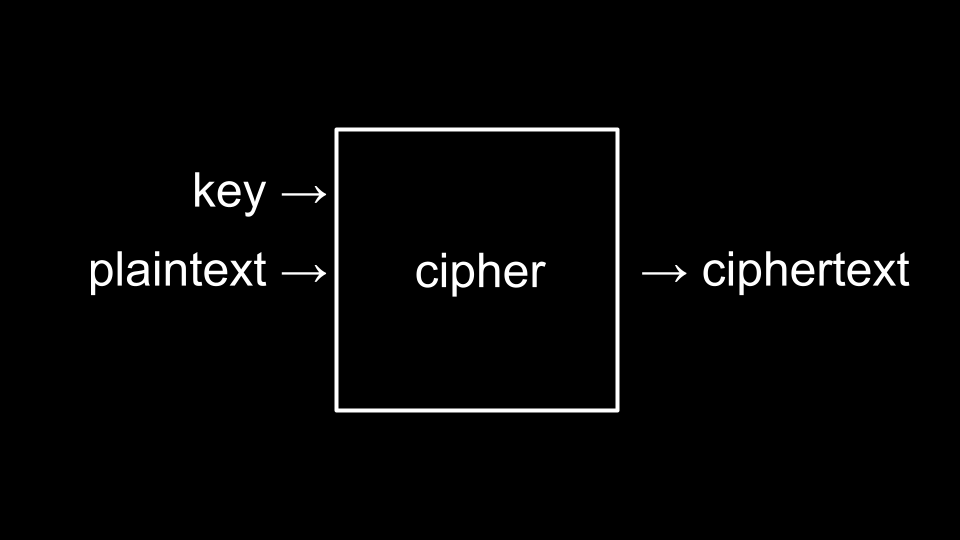• The key is a special argument passed to the cipher along with the plaintext. The cipher uses the key to make decisions about how to implement its cipher algorithm.

## Summing Up

In this lesson, you learned more details about compiling and how data is stored within a computer. Specifically, you learned…

• Generally, how a compiler works.
• How to debug your code using four methods.
• How to utilize arrays within your code.
• How arrays store data in back to back portions of memory.
• How strings are simply arrays of characters.
• How to interact with arrays in your code.
• How command-line arguments can be passed to your programs.
• The basic building-blocks of cryptography.

See you next time!